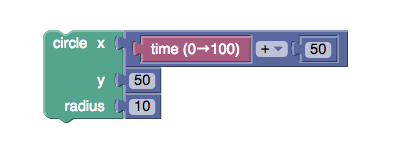# Animation Math

You don't need to know advanced math to program, but you need to know how to use basic math functions to communicate with a computer. To create computer graphics, use functions and precise numbers to instruct the computer what to draw.

Using the Math block to perform simple math operations. It will return the result of the operation. For example, in this block, the value of `x` will set to `time + 50`:Level Guidelines

3 . The 'time' block counts from 0 to 100. But this time you want the horizontal position of the red ball to start at 100 and go to 0. Can you figure out a simple mathematical formula that flips the direction?

4 . Create four circles on the four edges and make each circle move to its opposite side.

5 . It's straightforward to get the big circle to move correctly, but you'll need some math to move the small circles in the right location.

6 . These two lines have the same starting point, but you need to specify the motion of both their starting and ending points.

Optional Levels

7 . When programming, you sometimes are given a specific function you need to implement. You'll need to nest many math blocks together to write the function. (Once you switch to text-based programming, these lines become easier to write!)

Try to solve the harder levels 8 and 9 as well, and make any animation you want for level 10!

More games at Blockly Games